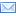#Difference Between Similar Terms and Objects

# Difference Between Speed and AccelerationSpeed vs Acceleration

Speed and acceleration are entirely different concepts. Every movable object is associated with motion which is measured in terms of speed. When an object starts moving, its speed is zero in the beginning, and it increases with time due to acceleration. If the body achieves a constant speed, then the acceleration ceases to exist.

Speed

Speed is defined as the rate of change of the position of a body. Thus it may be understood as distance covered by the body in a unit time. So speed may be expressed as:

Speed = Distance/Time

If “d” is the distance covered by a body in time “t,” then its speed “s” is:

s = d/t

If the distance is measured in meters and time in seconds, then the unit of speed will be meters per second.

Speed is a scalar quantity as it has only magnitude and not any specific direction. Related to speed is another term of velocity.

As already mentioned, speed has no specific direction and so it is a scalar quantity. If speed has specific direction, then it is termed as velocity. Thus velocity has magnitude as well as direction.

If a car is moving in a circle, it will have speed, but its velocity will be zero as there is no change of direction.

Acceleration

Acceleration is defined as the rate of change of velocity. So acceleration is the change in velocity per unit time. If a body has uniform velocity, its acceleration is zero. For a body to have acceleration, there must be a change in its velocity. Thus:

Acceleration = Velocity/Time

If “v” is the velocity of the body and “t” is the time taken by the body to achieve the velocity “v,” then its acceleration “a” may be understood as:

A = v/t

If the unit of velocity is in meters per second and the time is in seconds, then the unit of acceleration will be meters per second squared.

Taking the example of the car, if it is moving in a circle, the change in velocity is not there, but the direction of motion is changing and so acceleration is there.
Summary:

Speed is the distance covered in a unit of time while acceleration is the rate of change of speed.
The unit of speed in the metric system is meters per second (m/s) while that of acceleration is meters per second squared (m/s2).
Speed is a scalar quantity while acceleration is a vector quantity.
Speed is related to the rate of motion while acceleration is related to the rate of motion as well as the direction of motion.

### Search DifferenceBetween.net :

Custom Search

Help us improve. Rate this post!(3 votes, average: 4.67 out of 5)Loading...Email This Post : If you like this article or our site. Please spread the word. Share it with your friends/family.

1. Thanks! your wonderful website just helped me get my grade up in my science class!!! 🙂

• really nice

2. Awesome it helped me with a lot of confusion on hoe to differentiate between speed and acceleration

3. Thank for claering the concept of speed and velocity it really helps me thank qqq sooo much

4. Its a better example stead while fasing competitive exam

• You can even spell faced properly and you are doing an exam you are a disgrace

• You can’t even spell “can’t” and you’re mocking someone

6. thanks ,your websaite help me a lot.

7. Good

• LOL

8. Good very good and helpful

9. Great explanations

10. Thank you for the explanation

Please note: comment moderation is enabled and may delay your comment. There is no need to resubmit your comment.

Articles on DifferenceBetween.net are general information, and are not intended to substitute for professional advice. The information is "AS IS", "WITH ALL FAULTS". User assumes all risk of use, damage, or injury. You agree that we have no liability for any damages.